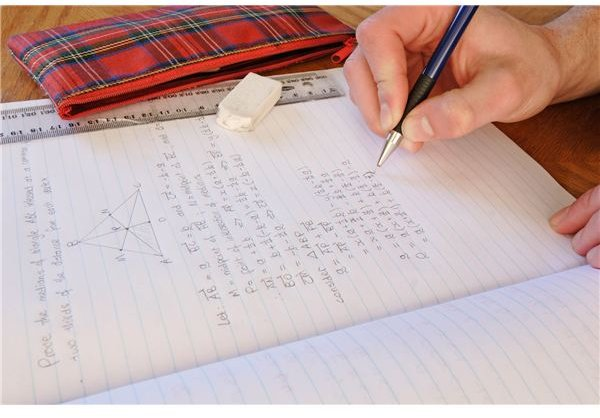# Learn How to Calculate an Average: Steps to Determine Average Rate of Change Plus Two Examples

Page contentThe concept of the average, related to the mode and the median, is one of the most powerful and simple mathematical calculations you will learn. The average, also known as the mean, is a useful way of summarizing performance, business activity, and other groups of numbers. After learning how to calculate an average, you can move on to other statistical concepts such as standard deviation. After you understand the concept, you can use statistics to make business decisions, solve scientific problems and other uses.

## Example 1: Calculate An Average At School

Teachers, administrators and students calculate averages all the time to measure progress and obtain a better understanding of the school.

Example 1: What Is the Average Test Score?

On Tuesday, nine students took a math test and received different scores. The students received a range of scores. The teacher wants to know what the average score is to determine whether the test was fair and to decide which students need extra math tutoring. The students received the following scores: 23, 41, 60, 69, 73, 79, 89, 95, and 100.

Go through these steps to calculate the average score:

• Find out how many scores there are. In this case, there are nine scores.
• Add up all of the scores: In this example, the total would be 629.
• Divide the total of the scores by the number of scores: 629 divided by 9 equals 69.888.

In many cases, the average would likely be rounded to the nearest whole number. If the average was rounded in this example, the average test score would be 70. In that case, the teacher knows that students who score under 70 are likely most in need of additional tutoring.

## Example 2: The Business World

Businesses, analysts, and many other professionals in the business world constantly calculate averages. One of the most popular uses for averages in business is to measure change over time. In the example in this section, we will look at how to calculate sales average.

Example 2: What is the average sales per salesperson?

Jane is a manager of an office supply company and has decided to give a \$1000 bonus to the sales person with the highest average sales in the last three months (also known as a quarter). Simply looking at the past three months of sales figures is overwhelming, so she decides to calculate an average.

January sales figures:

John sold 10 units, Anne sold 13 units and Toby sold 19 units.

February sales figures:

John sold 4 units, Anne sold 7 units, and Toby sold 6 units.

March sales figures:

John sold 23 units of supplies. Anne sold 31 units and Toby sold 25 units.

How To Calculate John’s average sales:

• How many months worth of sales are being averaged? 3 months
• How many sales were made in each month: 10, 4, and 23.
• What is the total of John’s sales?: 37
• What Is John’s average number of sales per month: 12.33 sales per month (total number of sales divided by the number of months, 37 / 3)

Before reading further, use the above steps to find out the average for the other sales staff.

Answer: John’s average was 12.33 sales per month, Anne’s average was 17 sales per month and Toby’s average was 16.66. Though it was a close decision, Jane decides to award the \$1000 bonus to Anne based on her sales.